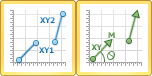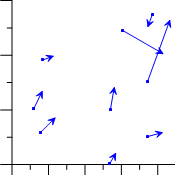# Vector Plots

 Click the Home | New Graph | Vector | 2-Point Vector Plot or Home | New Graph | Vector | 1-Point Vector Plot command to create a 2D vector plot. Vector plots create vector lines between two points or from one point to the specified angle direction and with the selected magnitude. Click the 2-Point Vector Plot or 1-Point Vector Plot button to create a vector plot.2-Point Vector Plots are created from four data columns. Two columns specify the starting X and Y location. Two other columns specify the ending X and Y location. A straight line with a vector arrow is drawn between the two points.

1-Point Vector Plots are also created from four data columns. Two columns specify the starting X and Y location. Two other columns specify the direction and magnitude of the vector. A straight line is drawn from the starting X and Y location in the direction specified with a length of the magnitude. The direction column is specified in degrees and increases in the clockwise direction, where 0 or 360 degrees is Up (North), 90 degrees is Right (East), 180 degrees is Down (South), and 270 degrees is Left (West).Vector plots show data asstraight-line segments.

## Creating a New Vector Plot

To create a vector plot:

1. Click the Home | New Graph | Vector | 2-Point Vector Plot or Home | New Graph | Vector | 1-Point Vector Plot command.
2. Select a worksheet file in the Open Worksheet dialog. You can select a new data file or you can select an open worksheet file in the Open worksheets section.
3. Click the Open button. A vector plot is created using the default properties.

## Editing Vector Plot Properties

To change the features of the vector plot, including the columns used to create the plot, first select the plot in the plot window or Object Manager and then edit its properties in the Property Manager. Click the following tabs in the Property Manager to change different properties: